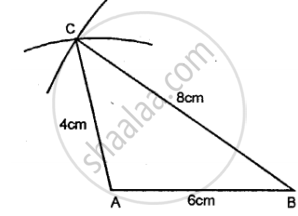# Construct traingle ABC, when : AB = 6 cm, BC = 8 cm and AC = 4 cm. - Mathematics

Diagram

Construct traingle ABC, when : AB = 6 cm, BC = 8 cm and AC = 4 cm.

#### Solution

Steps of Construction:

(1) Draw a line AB = 6 cm.(2) compasses and taking B as centre, draw an arc of 8 cm radius.

(3) With A as centre, draw an arc of 4 cm radius, which cuts the previous arc at C.

(4) Join AC and BC.

Triangle ABC, obtained, is the required triangle.

Concept: Classification of Triangles (On the Basis of Sides, and of Angles)
Is there an error in this question or solution?

#### APPEARS IN

Selina Class 6 Mathematics
Chapter 26 Triangles (Including Types, Properties and Constructions)
Exercise 26 (B) | Q 1